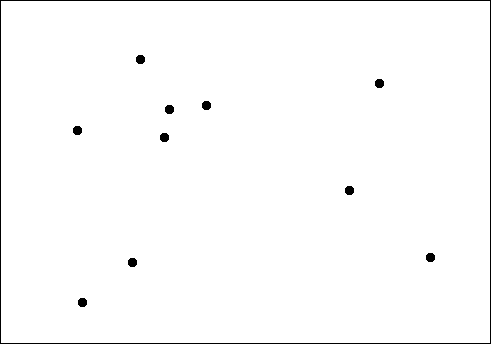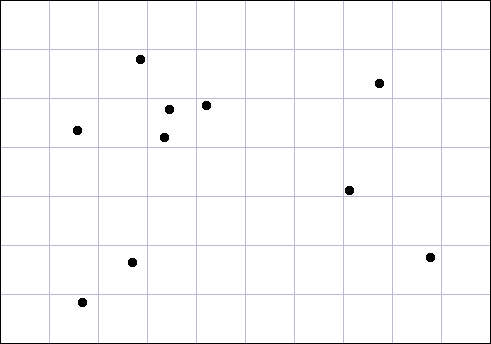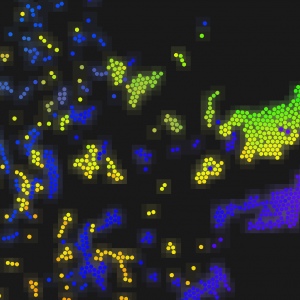Basic Spatial Hashing with Boost

So let’s say you’ve got some particles in 2-dimensional space.There they are. Now let’s say you want any two particles which are very close to each other to interact. You need to know which pairs are close enough. You could take all possible pairs of particles, calculate their distances, and compare those to the minimum distance d. That’s fine if you have 10 particles, but if you have 5,000 that’s going to add up to over 12,000,000 pairs. That’s too many pairs.

That complexity can be alleviated if for each particle P we can efficiently find a subset of all particles which includes all those within d of P and which includes as few as possible which aren’t within d. Then we can calculate the distances from P to the few particles in this subset and determine which ones are actually within distance d.

Enter: spatial hashing. I’ll show how boost::unordered_multimap can be used to do this.

The unordered_multimap is a key-value storage where multiple values are allowed to have the same key. Under the hood of this object is a hash table. This means that the type used for keys must have an equality operator and a hash function yielding an unsigned integer which is “very likely” to be unique.

Since each key in the multimap is ultimately reduced to a single integer, and what we’re trying to do is look up particles based on their positions, creating a hash function amounts to defining a mapping from continuous 2D space into discrete 1D space. A fine way to do this is to break down the continuous space by a grid and then combine the discrete grid coordinates into a single value with a generic hash function.There’s your grid. The resolution of this grid i.e. the size of the cells is not arbitrary. More on that later.

It doesn’t take very much code to implement the concepts so far:

 inline vec_2i cell ( const vec_2f & p, float cellsize ) { return vec_2i( boost::math::iround( p.x / cellsize ), boost::math::iround( p.y / cellsize ) ); }   inline size_t hash_value ( const vec_2i & v ) { size_t h = 0xdeadbeef; boost::hash_combine( h, v.x ); boost::hash_combine( h, v.y ); return h; }   typedef boost::unordered_multimap< vec_2i, particle_t > positionhash_t;
• The multimap value type could instead be a pointer or it could be an array index; it’s a data structure in this case for the sake of clarity. What’s best depends on what you’re up to.
• cellsize is the width and height of the grid cells in continuous space. The reason for keeping the real position to grid position conversion in a separate function rather than using the real position itself as the multimap key will become apparent.Let’s focus on the particle P at the center of the blue circle. The circle has radius d (the distance within which we want to know about other particles). If the grid cells are at least d on a side then all particles within the circle will be found in the nine shaded cells (the cell containing P and the eight surrounding cells). Now it becomes clear why we want to use grid coordinates as keys rather than real coordinates: the keys for neighboring cells can be determined easily by adding/subtracting 1 to/from the x and y components. So, cellsize must be at least d for this to be correct, but it need not be larger than d.

Optimally cellsize = d, this is what I’m getting at.

In this case the subset of particles we find includes two which are within d and one which is not; so we’ve got a 66% hit rate here. In general, if the particles are uniformly distributed, the hit rate of this spatial hashing process will be the circle’s area divided by the area of nine cells; with cellsize = d that’s roughly 35%.

OK then, let’s move on to determining the neighboring pairs of particles over the entire set of particles. If you insert all particles into the multimap and then examine each particle as we just did you’ll end up calculating all distances twice, and you’ll end up with two copies of each pairing. To refine this such that we don’t run redundant calculations, don’t produce redundant pairings, and don’t use any additional data structures, we can simply intermix the processes of inserting particles in the multimap and searching for neighboring particles.

To find each pair only once:

2. For each particle P
1. Determine which cell will contain P
2. Retrieve all particles in P‘s cell and the eight neighboring cells
3. Calculate distance from P to each of these and compare to d
4. Insert P into multimap

The code would look a little something like this:

 vec_2i offsets[ 9 ]; for( int dx = -1; dx <= 1; ++dx ) for( int dy = -1; dy <= 1; ++dy ) offsets[ (dx+1) + 3 * (dy+1) ] = vec_2i( dx, dy );   positionhash.clear(); BOOST_FOREACH( const particle_t & P, particles ) { for( size_t oi = 0; oi < 9; ++oi ) { std::pair< positionhash_t::const_iterator, positionhash_t::const_iterator > r = positionhash.equal_range( cell(P.pos, d) + offsets[oi] ); for( positionhash_t::const_iterator n = r.first; n != r.second; ++n ) { // if distance from P to n->second is less than d: // note P and n->second as a neighboring pair } }   positionhash.insert( positionhash_t::value_type( cell(P.pos, d), P ) ); }

And there you have it. I applied this technique in a toy particle simulation, and I was able to go from simulating around 300 particles to simulating 7,000 or more. Because the asymptotic complexities are different, the difference in efficiency between this spatial hashing approach and the naive quadratic approach grows larger as the number of particles increases.I have not tried applying this to 3 dimensions, and I have no plans to. It would not be difficult to adapt, but cost would increase:

• Real positions would have three components as would the grid positions. Hashing would be 150% as complex.
• Instead of 8 neighboring cells, there would be 26. Querying for potential neighboring particles would be 300% as complex.
• For uniformly distributed particles, hit rate would drop more than half. Testing potential neighboring particles would be at least 225% as complex.
This entry was posted in Uncategorized and tagged , , . Bookmark the permalink.